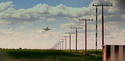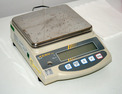Unit 5 Algebraic Reasoning

# Unit Description

In this unit, you will learn more about expressions and equations. You will write, evaluate, and simplify expressions that contain positive and negative numbers. You will write algebraic expressions and equations to represent situations, and you will solve these expressions and equations using formal algebraic methods and number properties. You will use estimation and mental math to find an approximate solution. Finally, you will learn how linear inequalities are different from linear equations. You will use inequalities to represent real-life situations, and find solutions to the problems by writing and solving inequalities.

•••••••••Lesson 9

Peer Review`
•••••••